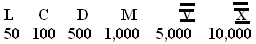Name: ___________________Date:___________________

 Email us to get an instant 20% discount on highly effective K-12 Math & English kwizNET Programs!

### Grade 3 - Mathematics13.1 Roman Numerals

Table for Roman Numerals
 Roman Numerals I V X L C D M Indo Arabic Numerals 1 5 10 50 100 500 1000
Rules:
1. If a symbol is repeated twice or thrice, the value of the numeral is obtained by adding the values of the symbol as many times as it is repeated.
Examples:
II = 1+1 = 2
III = 1+1+1 =3
XX = 10+10 = 20
Note: The symbol V is never repeated.
2. To write a number in which the smallest digit always comes to the right of the greater digit, we add the values of all the digits.
Examples:
VII = 5 + 1 + 1 = 7
XI = 10 + 5 = 15
LXVI = 50 + 10 + 5 +1 = 66
3. To write a number in which the smaller digit is placed before the greater digit, we subtract the value of the smaller digit from that of the greater digit.
Examples:
IV = 5 - 1 = 4
LIX = 50 + (10-1) = 59
Note: The symbol V is never subtracted.
4. For the number beyond 10, we first write the number in groups of 10s and Is and then form the Roman numeral corresponding to the given number.
Examples:
12 = 10+2 = XII
20 = 10+10 = X+X = XX
24 = 10+10+4 = 10+10+(5-1) = X+X+IV = XXIV
26 = 10+10+6 = XXVI
39 = 10+10+10+9 = XXXIXDirections: Answer the following questions. Also write a table for roman numerals from 1 - 40 and ten examples of your own.Name: ___________________Date:___________________

### Grade 3 - Mathematics13.1 Roman Numerals

 Q 1: The Roman Numerals areI II III IV V VI VII VIII IX X0 1 2 3 4 5 6 7 8 9 Q 2: What is the modern equivalent of Roman number X?51050 Q 3: What is the modern equivalent of Roman number L?604050 Q 4: What is the modern equivalent of Roman number C?10100500 Q 5: What is the modern equivalent of Roman number LXXX?807060 Q 6: The numerals we use most often are0 1 2 3 4 5 6 7 8 9I II III IV V VI VII VIII IX X Question 7: This question is available to subscribers only! Question 8: This question is available to subscribers only!

#### Subscription to kwizNET Learning System costs less than \$1 per month & offers the following benefits:

• Unrestricted access to grade appropriate lessons, quizzes, & printable worksheets
• Instant scoring of online quizzes
• Progress tracking and award certificates to keep your student motivated
• Unlimited practice with auto-generated 'WIZ MATH' quizzes